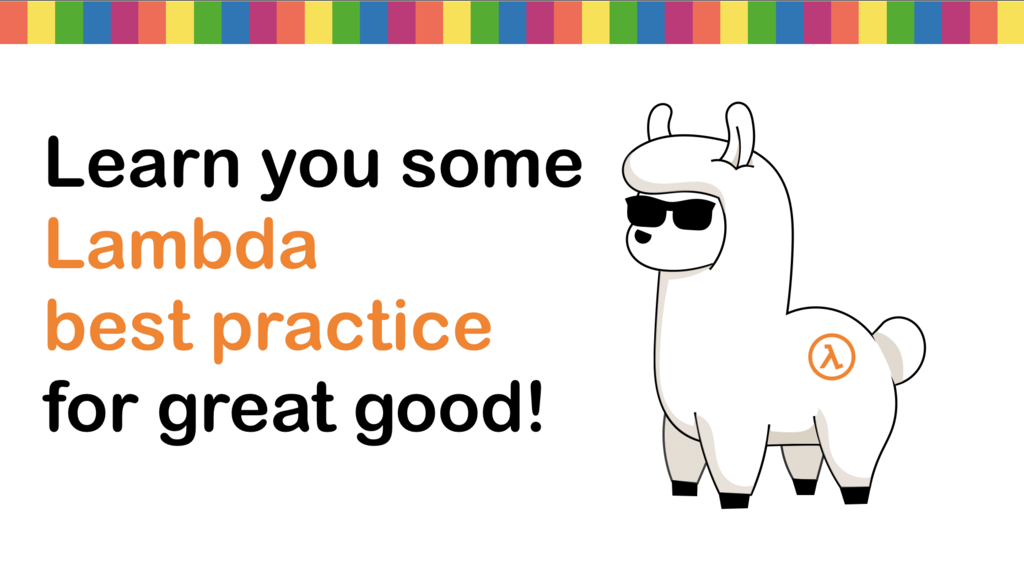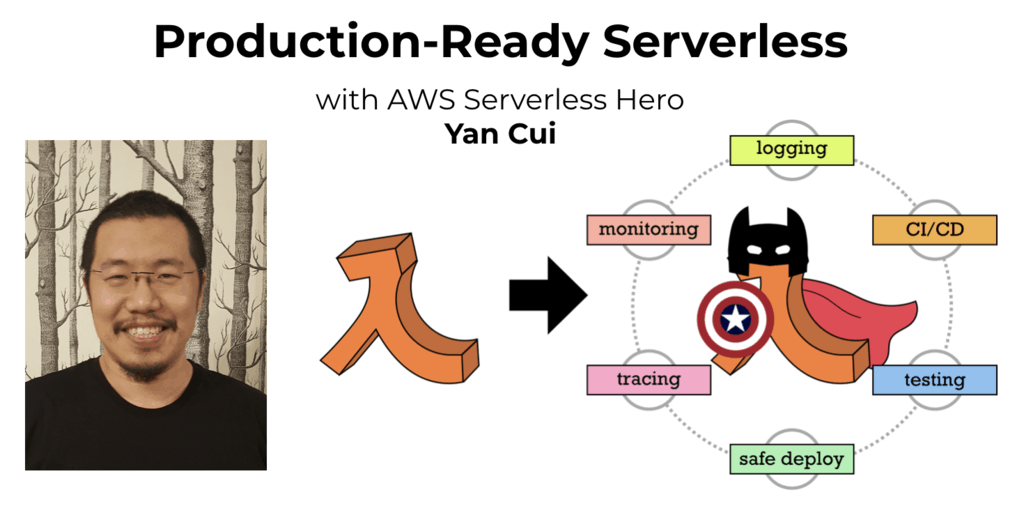# Project Euler – Problem 25 Solution

Check out my new course Learn you some Lambda best practice for great good! and learn the best practices for performance, cost, security, resilience, observability and scalability.

#### Problem

The Fibonacci sequence is defined by the recurrence relation:

Fn = Fn-1 + Fn-2, where F1 = 1 and F2 = 1.

Hence the first 12 terms will be:

F1 = 1

F2 = 1

F3 = 2

F4 = 3

F5 = 5

F6 = 8

F7 = 13

F8 = 21

F9 = 34

F10 = 55

F11 = 89

F12 = 144

The 12th term, F12, is the first term to contain three digits.

What is the first term in the Fibonacci sequence to contain 1000 digits?

#### Solution

```let fibonacciSeq =
Seq.unfold (fun (current, next) -> Some(current, (next, current + next))) (0I, 1I)
|> Seq.filter (fun f -> f > 0I)

let answer = (Seq.findIndex (fun f -> f.ToString().Length >= 1000) fibonacciSeq) + 1
```

In my solution here I first created a Fibonacci sequence which starts with 1, 1, … all the way to infinity, and the second expression gives us the answer to the problem by using Seq.findIndex to find the index (zero-based) of the first element which contains 1000 digits. The ‘+ 1’ at the end simply converts the zero-based index to the one-based index which the problem is asking for.Hi, I’m Yan. I’m an AWS Serverless Hero and the author of Production-Ready Serverless.

I specialise in rapidly transitioning teams to serverless and building production-ready services on AWS.

Are you struggling with serverless or need guidance on best practices? Do you want someone to review your architecture and help you avoid costly mistakes down the line? Whatever the case, I’m here to help.Check out my new course, Learn you some Lambda best practice for great good! In this course, you will learn best practices for working with AWS Lambda in terms of performance, cost, security, scalability, resilience and observability. Enrol now and enjoy a special preorder price of £9.99 (~\$13).Are you working with Serverless and looking for expert training to level-up your skills? Or are you looking for a solid foundation to start from? Look no further, register for my Production-Ready Serverless workshop to learn how to build production-grade Serverless applications!